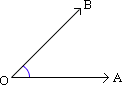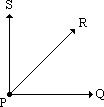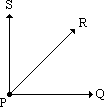## Definition Of Angle

An angle is formed by two rays with a common endpoint (called the vertex).

### Examples of Angle

• In the figure shown, angle AOB is formed by the rays OA and OB with a common endpoint O.### Solved Example Clockple on Angle

#### Ques: How many angles are formed by three rays with a common vertex?#### Choices:

A. 3
B. 2
C. 1
D. 4